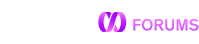# Median as an aggregate function

In Oracle I can do something like this:

SELECT COLUMN1, COLUMN2, SUM(COLUMN3), AVG(COLUMN3), MEDIAN(COLUMN3) FROM TABLE
GROUP BY COLUMN1, COLUMN2

Is there a way to achieve the same thing in MEMSQL?
I tried PERCENTILE_CONT, but the best I could get is the median group by COLUMN1 and COLUMN2, and I don’t know how to fit SUM(COLUMN3) and AVG(COLUMN3) into the query.
I also tried create a custom function using CREATE AGGREGATE but have no luck.

Any suggestions?
Thanks.

You can use percentile_cont, but note that it is a window function and not supported as an aggregate function.

Example:

``````select sum(column3), avg(column3), any_value(median) from
(select column1, column2, column3, percentile_cont(0.5) within group (order by column3) over (partition by column1, column2) as median
from t) sub
group by column1, column2
``````

Here’s another variation of that idea using percentile_disc (to get a discrete median, not an interpolated one). It uses rank = 1 to pick out one row from the window.

``````create table t (g int, x int);
insert t values (1, 1), (1, 2), (1, 3), (1, 4), (1,5);
insert t values (2, 1), (2, 2), (2, 3), (2, 4), (2,5);

with ranked as (
select g,
rank() over w as r,
percentile_disc(0.5) over w as median,
avg(x) over w as win_avg
from t
window w as (partition by g order by x
rows between unbounded preceding and unbounded following)
)
select * from ranked where r = 1;

+------+---+--------+---------+
| g    | r | median | win_avg |
+------+---+--------+---------+
|    1 | 1 |      3 |  3.0000 |
|    2 | 1 |      3 |  3.0000 |
+------+---+--------+---------+
``````

Your example doesn’t work for me. I am getting an error running the code exactly as you have it. The error I receive is: ERROR 1706 ER_MEMSQL_FEATURE_LOCKDOWN: Feature ‘Ordered aggregate window functions with named windows. Fix by defining the window inline.’ is not supported by MemSQL.

I could not find any documentation on this error. What is causing it?

The way I got your query to run was to alter the percentile function to not use the shared window but define an inline one. I still don’t understand the cause for the error though.

percentile_disc(0.5) over(partition by g order by x rows between unbounded preceding and unbounded following) as median